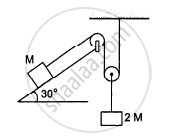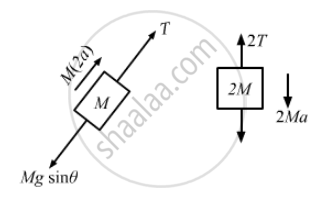# Find the Acceleration of the Block of Mass M in the Situation Shown in Figure (5−E15). All the Surfaces Are Frictionless and the Pulleys and the String Are Light. - Physics

Sum

Find the acceleration of the block of mass M in the situation shown in the following figure. All the surfaces are frictionless and the pulleys and the string are light.#### Solution

The free-body diagram of the system is shown below:Let acceleration of the block of mass 2M be a.
So, acceleration of the block of mass M will be 2a.

M(2a) + Mgsinθ − T = 0
⇒ T = 2Ma + Mgsinθ    ...(i)
2T + 2Ma − 2Mg = 0
From equation (i),
2(2Ma + Mgsinθ) + 2Ma − 2Mg = 0
4Ma + 2Mgsinθ + 2Ma − Mg = 0
6Ma + 2Mgsin30° + 2Mg = 0
6Ma = Mg
$\Rightarrow a = \frac{g}{6}$
Hence, the acceleration of mass
$M = 2a = 2 \times \frac{g}{6} = \frac{g}{3} \left (\text{ up the plane }\right) .$

Concept: Newton’s Second Law of Motion
Is there an error in this question or solution?

#### APPEARS IN

HC Verma Class 11, Class 12 Concepts of Physics Vol. 1
Chapter 5 Newton's Laws of Motion
Q 32 | Page 81